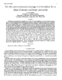## On the communication-storage minimization for a class of secure multicast protocols##### Files
CS-TR-4170.ps(492.59 KB)
Developing cryptographic key management protocols that have scalability in terms of the key storage as well as key update communication is an important problem in many secure multicast applications~\cite{rb1,dea,wgl}. Wong {\em et al.}~\cite{wgl} and Wallner {\em et al.}~\cite{dea} independently presented the first set of key distribution models where the key update communication grows as ${\cal O}(\log N)$ for group of size $N$. However, the storage requirement of these models were ${\cal O(N)$. Recently~\cite{cmn}, a new model based on clustering of the group members was proposed in order to lower the key storage while maintaining the update communication growth as ${\cal O}(\log N)$. For the new model, by considering the product of the storage and the communication as the cost function, the optimal cluster size $M$ was conjectured to be $M= {\cal O}(\log N)$. In this paper, we show that the optimal value of the cluster can be computed without the product function due the monotonicity of the storage with respect to the cluster size. We show that the optimal cluster size selection of the model in~\cite{cmn} can be formulated as a constraint optimization problem, and then transform it to a fixed point equation of the form $M - \lambda \log_e M = (\beta_2 - \lambda)\log_e N$, where $\beta_2, \lambda$ are model parameters. We first show that the largest root of this equation is the optimal solution, and then compute it by two different techniques. We then show that the first order approximation of the solution is of the form $M \approx (\beta_2 -\lambda)\log_e N + \lambda \log_e \log_e N$, leading to $M \approx (\beta_2 - \lambda) \log_e N$ for large values of $N$. We make a case for use of the estimate $M = (\beta_2 -\lambda) \log_e N + \lambda \log_e \log_e N$ instead of $M = \log_e N$ by showing that even for group size up to $2^{32}$, the value $M = \log_e N + \lambda \log_e \log_e N$ provides significantly lower value of key storage compared to the value $M = \log_e N$. We also show that the best estimate of $M$ using the product function in~\cite{cmn} does not exceed $M = \nu \log_e N$ for a constant $\nu$. (Also cross-referenced as UMIACS-TR-2000-58)